Win up to 100% scholarship on Aakash BYJU'S JEE/NEET courses with ABNAT Win up to 100% scholarship on Aakash BYJU'S JEE/NEET courses with ABNAT

# Circular Motion

## What Is Circular Motion?

Circular motion is described as a movement of an object while rotating along a circular path. Circular motion can be either uniform or non-uniform. During uniform circular motion, the angular rate of rotation and speed will be constant, while during non-uniform motion the rate of rotation keeps changing.

Some of the most common examples of circular motion include man-made satellite that revolves around the earth, a rotating ceiling fan, a moving car’s wheel, the blades in a windmill, and gears in gas turbines.

A particle is said to execute circular motion when it moves along the circumference of a circular path. An important aspect of circular motion is that the direction of motion is changing continuously unlike in the case of linear motion. Hence, circular motion can also be described in terms of angular variables.

## Angular Variables

Angular Displacement

It is defined as the angle turned by a rotating particle per unit time. It is represented by ∆θ and measured in radians. In the figure, angular displacement is measured between the position vectors rand r.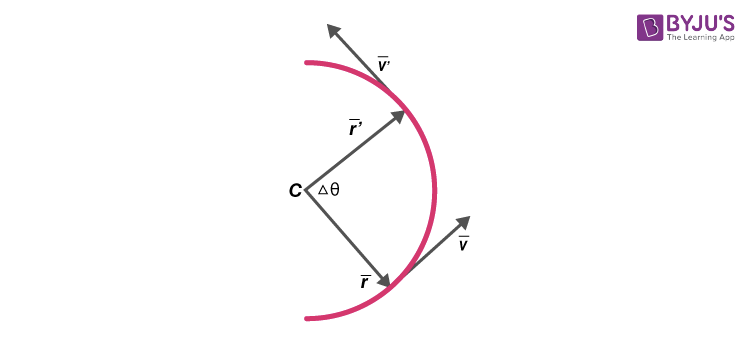Angular Velocity

It is defined as the rate of change in angular displacement of a particle in a circular motion. It is denoted by

ω = lim∆t→0 (∆θ/∆t) = dθ/dt

Angular velocity is measured in rad/s. Apart from angular velocity and angular speed, a particle in circular motion also possesses linear velocity and corresponding linear speed.

v= ds/dt

v= |ds/dt|; s is the displacement of the particle

### Relation between Linear Speed(V) and Angular Speed(Ω)

In vector form

v= ω x r

Where r is the position vector of the particle measured with respect to the centre of the circle.

(Or)

v = rω

The acceleration of a particle in circular motion has two components:

• Tangential acceleration at: This is the component of acceleration in the direction of the velocity of the particle.

at= d|v|/dt

• Radial acceleration ar: This is the component of acceleration directed towards the centre of the circle. This component causes a change in the direction of the velocity of a particle in a circular motion.

ar= v2/r = rω2

Angular Acceleration

It is defined as the rate of change of angular velocity of the rotating particle. It is measured in rad/s2

α = dω/dt = d2θ/dt2

Circular motion can be uniform and non-uniform depending on the nature of acceleration of the particle. The motion is called uniform circular motion when the particle is moving along a circular path possessing a constant speed.

During circular motion, the velocity vector changes its direction at each point on the circle. This implies that the radial component of acceleration is always non-zero. The tangential component can take a positive or negative value in the case of non-uniform circular motion and a zero value in the case of uniform circular motion.

We understand that the acceleration of a particle in a circular motion is always directed towards the centre and is given by v2/r. Applying Newton’s second law of motion in this situation;

Fcen = mv2/r

Where m is the mass of the particle

Fc is the centripetal force directed towards the centre of the circular path as illustrated in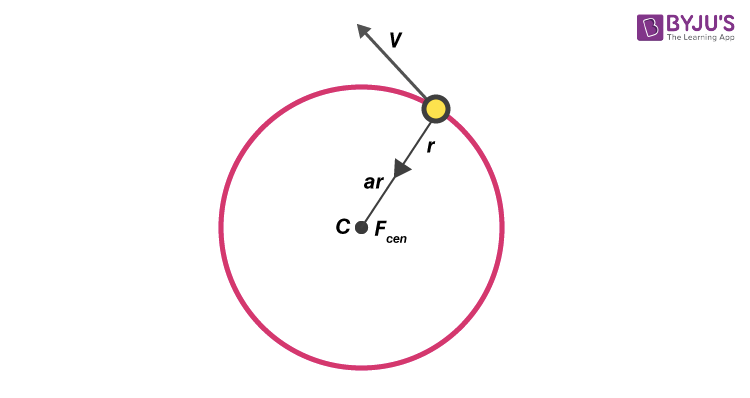Examples of centripetal forces include gravitational force, the tension in a string and friction.

## Application of Newton’s Laws of Motion

Let us understand the application of Newton’s laws of motion in two different scenarios. The motion of a vehicle will be on either:

At an instant when a vehicle makes a turn on a level road, there are three different forces acting.

1. The weight of the car W= mg
2. Frictional force ff
3. Normal reaction N

In the vertical direction N-mg = 0

N = mg

The centripetal acceleration for circular motion is provided by static friction. This friction holds back the vehicle from moving away from the circle.

ff = mv2/r ≤ µN

µis the coefficient of static friction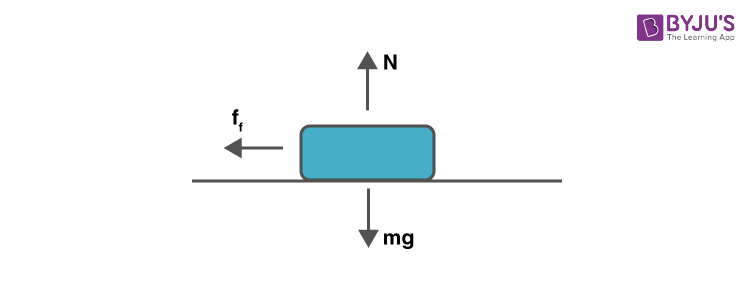$$\begin{array}{l}v_{max} = \sqrt{\mu rg}\end{array}$$

This implies that for a circular path of given radius and µ, there is a maximum possible speed of the vehicle.

The effect of friction on the motion of a vehicle on a circular road can be minimised if the road is slightly raised on the outer end. This is called banking. Let the road be banked at an angle θ0 as illustrated in the figure.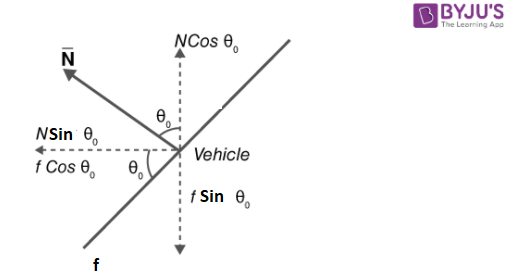Net force along the vertical direction is zero since there is no acceleration along this direction.

Therefore,

N cos θ0 = f sin θ0 + mg …..(1)

The centripetal force is provided by the horizontal component of N and f

mv2/r = N sin θ0 + f cos θ0….(2)

Substituting f= µN in equations (1) and (2) gives vmax

N cos θ0 = µN sin θ0 + mg

mv2/r = N sin θ0 + µN cos θ0

Solving the above equations gives us,

$$\begin{array}{l}v_{max} = \sqrt{rg\,\,\tan \,{{\theta }_{0}}}\end{array}$$

tan θ0 = vmax2/rg

## Circular Motion Videos

### Uniform Circular Motion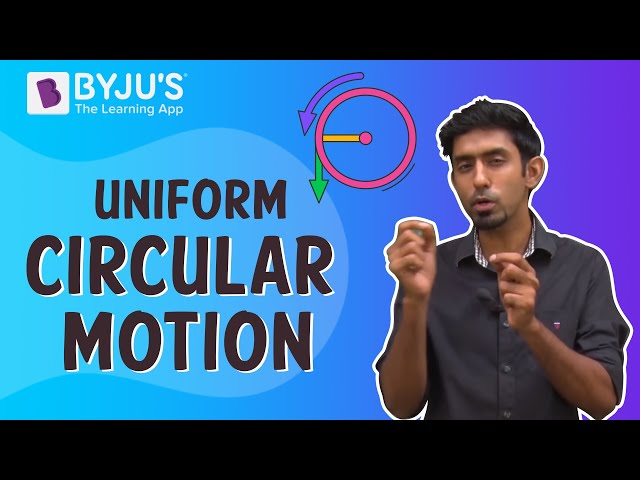### Radius of Curvature- Circular Motion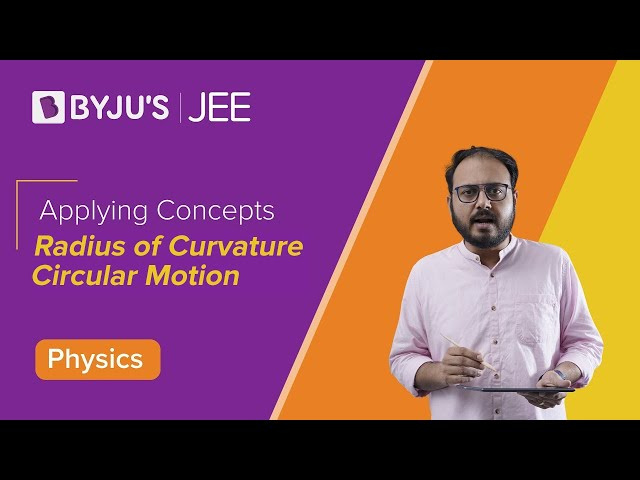### Non-Uniform Circular Motion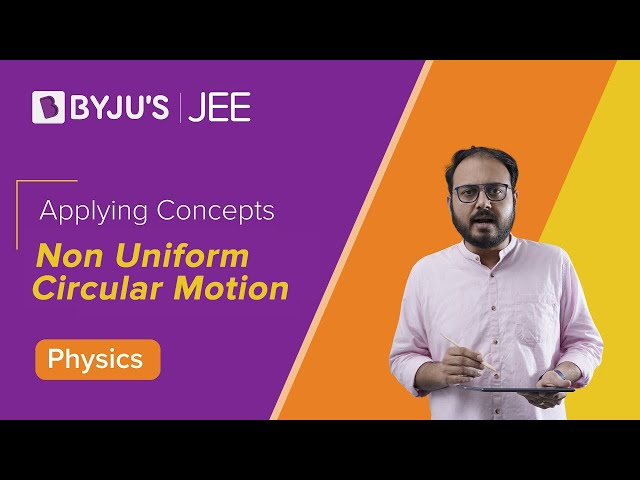### Application of Circular Motion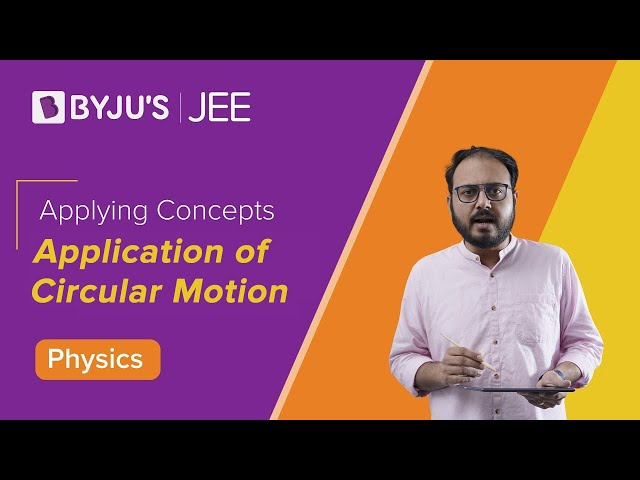## Solved Problems

Question 1: What is the angular velocity of a particle in a circular orbit, rotating at the rate of 200 times per minute?

Solution: In the given problem, the frequency of rotation is 200 times per minute

ω= 2πf

= 2π x 200/60 = 20.93 rad/s

Question 2: An electrically powered wheel is rotating about its axis at the rate of 900 rotations per minute. When the power is turned off it comes it rest in 1 minute. Find the angular deceleration of the wheel in rad/s2.

Solution: From the laws of motion in the case of rotational motion, We know that ω = ω0 + αt.

From the given data :

ω0 = 900rpm = 900 x 2π/60 rad/s

ω = 0 and t= 60s

Hence, angular deceleration α = π/2

Question 3: A bus is moving in a circular track of radius 1000cm with a speed of 10m/s. A pendulum is suspended from the roof of the bus by a light rigid rod of length 100cm. Find the angle made by the rod with the track. (Use g=10m/s2).

Solution:

tan θ = v2/rg = 102/10×10

tan θ = 1

tan θ = 45⁰

Question 4: The minimum velocity with which a car driver must traverse a curve of radius 150 m and coefficient of friction 0.6 to avoid skidding is? [IIT-JEE 2002].

Solution: In the relation mv2/r =µN substitute N = mg

We get v2 =µrg

v2 = 0.6x150x10

v= 30m/s

Question 5: Which of the following statements is false for a particle moving in a circle with a constant angular speed? [AIEEE 2004]

a)The velocity vector is tangent to the circle

b) The acceleration vector is tangent to the circle

c) The acceleration vector points to the centre of the circle.

d) The velocity and acceleration vectors are perpendicular to each other.

Solution: Option b)

## Frequently Asked Questions on Circular Motion

Q1

### What type of motion is called circular motion?

The motion of an object in a circular path along the circumference of a circle or rotation along a circular path is called circular motion.

Q2

### What are the types of circular motion?

Circular motion can be either uniform or non-uniform.

Q3

### Give an example of circular motion.

The movement of the satellite in a circular orbit, ice skaters going in a circle with constant velocity, and cars moving in a circular path are examples of circular motion.

Q4

### What is the value of instantaneous acceleration in a uniform circular motion?

The instantaneous acceleration in a uniform circular motion is given by
a = v2/R

v is the velocity of the object.
R is the radius of the circle.

The direction is perpendicular to velocity and directed inwards along the radius.

Test your Knowledge on Circular Motion